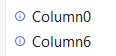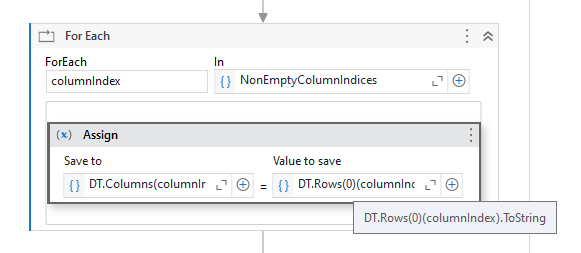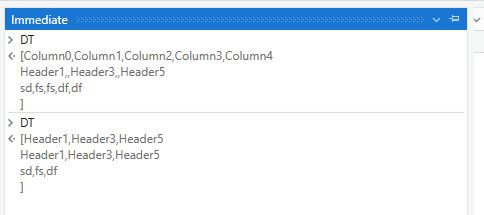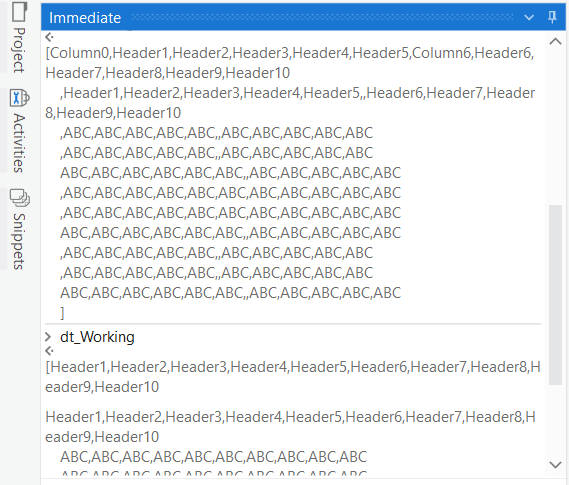# Remove column if row value is empty

I have this kind of data and I want to remove the whole column as the yellow part is empty.

The output should be like this. I cannot filter remove column as the column that should be deleted is in different each time.

I do this to remove column if all rows empty. But I don’t know how to modify it to my current need.

@arina

Loop through columns and check if there are empty rows

Initialize a counter =0

For each on enumerable.Range(0,dt.Columns.Count).ToArray and change type argument to integer

Then insde that use filter datatable and filter the dt with counter is empty

If condition with if filtereddt.rowcount>0

On then side use remove column and on else side use counter=counter+1

This will check each column if wmpty rows are there it deletes

Cheers

Hi @arina,

If you want to just remove the columns not containing the headers, then you can read it as datatable and put the following in an assign statement to remove the columns you do not require,

Hi @arina ,

`dt=dt.Select("Column0<>'' or Column8<>=''").CopyToDataTable()`

Regards,
Arivu

Would you mind explain a bit more on this? I able to get the column name but using activity filter data table and remove data column gave me error.test.xlsx (9.7 KB)

@arina

If you use dt.columns obviously you will get error…that is the reason i gave the solution with counter and looping through columns using the column number

The issue in your code is that in for loop you cannot manipulate the datatable if that datatable is used in the in arguments…

Cheers

Hi @arina ,

Could you check with the below steps :

1. Firstly, The Decision of preserving the Columns is decided based on the First Row. Hence we would need to identify which column indices there are non-empty values and preserve only them. For this we can use the below Expression to get the column Indices where the value is Empty for the first row data.
``````NonEmptyColumnIndices = DT.Rows(0).ItemArray.SelectMany(Function(x,i)if(String.IsNullOrWhiteSpace(x.ToString),{},{i})).ToArray
``````

Here, `DT` is the input datatable and `NonEmptyColumnIndices` is a variable of type Integer Array.

1. Next, we can rename the column names for only these identified column indices using a `For Each` Activity like shown below :In the assign activity , we have the below expression :
``````DT.Columns(columnIndex).ColumnName = DT.Rows(0)(columnIndex).ToString
``````
1. Next, we Filter out the remaining columns and keep only the columns required using the below expression :
``````DT = DT.DefaultView.ToTable(false,NonEmptyColumnIndices.Select(Function(x)DT.Columns(x).ColumnName).ToArray)
``````

Visuals :Let us know if you are able to get the expected result

Thank you !! I able to follow and visualize it well.1 Like

This topic was automatically closed 3 days after the last reply. New replies are no longer allowed.